# 2.2：使用通用策略求解线性方程

•• OpenStax
• OpenStax
$$\newcommand{\vecs}{\overset { \rightharpoonup} {\mathbf{#1}} }$$ $$\newcommand{\vecd}{\overset{-\!-\!\rightharpoonup}{\vphantom{a}\smash {#1}}}$$$$\newcommand{\id}{\mathrm{id}}$$ $$\newcommand{\Span}{\mathrm{span}}$$ $$\newcommand{\kernel}{\mathrm{null}\,}$$ $$\newcommand{\range}{\mathrm{range}\,}$$ $$\newcommand{\RealPart}{\mathrm{Re}}$$ $$\newcommand{\ImaginaryPart}{\mathrm{Im}}$$ $$\newcommand{\Argument}{\mathrm{Arg}}$$ $$\newcommand{\norm}{\| #1 \|}$$ $$\newcommand{\inner}{\langle #1, #2 \rangle}$$ $$\newcommand{\Span}{\mathrm{span}}$$ $$\newcommand{\id}{\mathrm{id}}$$ $$\newcommand{\Span}{\mathrm{span}}$$ $$\newcommand{\kernel}{\mathrm{null}\,}$$ $$\newcommand{\range}{\mathrm{range}\,}$$ $$\newcommand{\RealPart}{\mathrm{Re}}$$ $$\newcommand{\ImaginaryPart}{\mathrm{Im}}$$ $$\newcommand{\Argument}{\mathrm{Arg}}$$ $$\newcommand{\norm}{\| #1 \|}$$ $$\newcommand{\inner}{\langle #1, #2 \rangle}$$ $$\newcommand{\Span}{\mathrm{span}}$$$$\newcommand{\AA}{\unicode[.8,0]{x212B}}$$

##### 摘要

• 使用交换和关联属性
• 使用恒等式、反向和零的属性
• 使用分布属性简化表达式

1. 简化：$$\frac{3}{2}(12x+20)$$
2. 简化：$$5−2(n+1)$$
3. 找到$$\frac{5}{6}$$和的液晶屏$$\frac{1}{4}$$

## 使用一般策略求解线性方程

##### 确定一个数字是否是方程的解
1. 用数字代替方程中的变量。
2. 简化方程两边的表达式。
3. 确定所得方程是否正确。
• 如果是真的，那么这个数字就是一个解。
• 如果不是，则该数字不是解决方案。
##### 示例$$\PageIndex{1}$$

1. $$y=\frac{3}{5}$$
2. $$y=\frac{7}{5}$$

 $$5 y+3=10 y-4$$ 替代$$\color{rec}\frac{3}{5}$$$$y$$ $$5\left( \color{red} \frac{3}{5} \color{black}\right)+3 \stackrel{?}{=} 10\left( \color{red}\frac{3}{5} \color{black}\right)-4$$ 乘。 $$3+3\stackrel{?}{=} 6-4$$ 简化。 $$6 \neq 2$$

b。

 $$5 y+3=10 y-4$$ 替代$$\color{red} \frac{7}{5}$$$$y$$ $$5\left(\color{red} \frac{7}{5} \color{black}\right)+3 \stackrel{?}{=} 10\left(\color{red}\frac{7}{5}\color{back}\right)-4$$ 乘。 $$7+3 \stackrel{?}{=} 14-4$$ 简化。 $$10=10 \checkmark$$

##### 练习$$\PageIndex{1A}$$

1. $$y=\frac{4}{3}$$
2. $$y=\frac{1}{3}$$

##### 练习$$\PageIndex{1B}$$

1. $$x=\frac{3}{2}$$
2. $$x=−\frac{1}{2}$$

##### 线性方程

$ax+b=0$

##### 示例$$\PageIndex{2}$$$$m=2$$

##### 练习$$\PageIndex{2B}$$

$$a=0$$

##### 使用一般策略求解线性方程
1. 尽可能简化方程的每一面。
使用分布属性删除所有括号。
将相似的术语组合在一起。
2. 收集方程一侧的所有变量项。

使用等式的加法或减法属性。

3. 收集方程另一侧的所有常量项。

使用等式的加法或减法属性。

4. 使变量项的系数等于 1。

使用等式的乘法或除法属性。

陈述方程的解。

5. 检查解决方案。

将解替换为原始方程以确保结果是真实的陈述。

##### 示例$$\PageIndex{3}$$

 $$\frac{2}{3}(3 m-6)=5-m$$ 分发。 $$2 m-4=5-m$$ $$m$$将两边相加，只得到左边的变量。简化。 $$3 m-4=5$$ $$4$$将两边相加，只获得右边的常量。简化。 $$3 m=9$$ 将两边除以三。简化。 $$m=3$$
 查看：让$$m=3$$。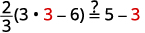##### 练习$$\PageIndex{3A}$$

$$u=2$$

##### 练习$$\PageIndex{3B}$$

$$x=4$$

##### 示例$$\PageIndex{4}$$

 $$4(x-1)-2=5(2 x+3)+6$$ 分发。 $$4 x-4-2=10 x+15+6$$ 将相似的术语组合在一起。 $$4 x-6=10 x+21$$ $$4x$$从两边减去以后只能得到右边的变量$$10>4$$。 $$4 x \color{red} -4 \color{black} x-6=10 x \color{red}-4 x \color{black}+21$$ 简化。 $$-6=6 x+21$$ $$21$$从两边减去得到左边的常量。 $$-6 \color{red} -21 \color{black} =6 x+21 \color{red}-21$$ 简化。 $$-27=6 x$$ 将两边除以$$6$$。 $$\frac{-27}{\color{red}6} \color{black}=\frac{6 x}{\color{red}6}$$ 简化。 $$-\frac{9}{2}=x$$ 查看： $$4(x-1)-2=5(2 x+3)+6$$\) 让$$x=−92$$。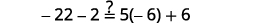##### 练习$$\PageIndex{4A}$$

$$p=−2$$

##### 练习$$\PageIndex{4B}$$

$$q=−8$$

##### 示例$$\PageIndex{5}$$

 $$10[3-8(2 s-5)]=15(40-5 s)$$ 首先从最里面的圆括号中进行简化。 $$10[3-16 s+40]=15(40-5 s)$$ 在方括号中组合相似的术语。 $$10[43-16 s]=15(40-5 s)$$ 分发。 $$430-160 s=600-75 s$$ $$160s$$将两边相加，$$160s$$使两边都加上，使变量向右。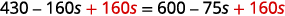简化。 $$430=600+85 s$$ $$600$$从两边减去将常量放在左边。简化。 $$-170=85 s$$ 将两边除以$$85$$。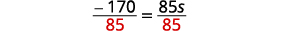简化。 $$-2=s,$$所以$$s = -2$$ 查看： $$10[3-8(2 s-5)]=15(40-5 s)$$ 让$$s=−2$$。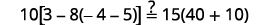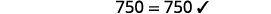##### 练习$$\PageIndex{5A}$$

$$y=−\frac{17}{5}$$

##### 练习$$\PageIndex{5B}$$

$$z=0$$

## 对方程进行分类

##### 条件方程

 $$7 y+14=7(y+2)$$ 分发。 $$7 y+14=7 y+14$$ 减$$7y$$去每一边，使$$y’$$ s变为一边。 $$7 y \color{red}-7 y \color{black} +14=7 y \color{red} -7 y \color{black}+14$$ 简化 —$$y$$ — 被淘汰了。 $$14=14$$ 但$$14=14$$确实如此。

##### 身份

 $$-8 z=-8 z+9$$ 两边$$8z$$相加，将常数单独留在右边。 $$-8 z \color{red} +8 z \color{black}=-8 z \color{red}+8 z \color{black} +9$$ 简化 —$$z$$ — 被淘汰了。 $$0 \neq 9$$ 但是$$0≠9$$。

##### 示例$$\PageIndex{6}$$

 $$6(2 n-1)+3=2 n-8+5(2 n+1)$$ 分发。 $$12 n-6+3=2 n-8+10 n+5$$ 将相似的术语组合在一起。 $$12 n-3=12 n-3$$ $$12n$$从两边减去，得到$$n$$ s 为一边。简化。 $$-3=-3$$ 这是真实的陈述。 方程是一个恒等式。 解决方案都是实数。

##### 示例$$\PageIndex{7}$$

 $$8+3(a-4)=0$$ 分发。 $$8+3 a-12=0$$ 将相似的术语组合在一起。 $$3 a-4=0$$ $$4$$添加到两边。 $$3 a-4 \color{red}+4 \color{black}=0 \color{red}+4$$ 简化。 $$3 a=4$$ 除以。 $$\frac{3 a}{\color{red}3} \color{black}=\frac{4}{\color{red}3}$$ 简化。 $$a=\frac{4}{3}$$ 等式在以下情况下是正确的$$a=\frac{4}{3}$$。 这是一个条件方程。 解决方案是$$a=\frac{4}{3}$$。

##### 示例$$\PageIndex{8}$$

 $$5 m+3(9+3 m)=2(7 m-11)$$ 分发。 $$5 m+27+9 m=14 m-22$$ 将相似的术语组合在一起。 $$14 m+27=14 m-22$$ $$14m$$从两边减去。 $$14 m+27 \color{red}-14 m \color{black}=14 m-22 \color{red}-14 m$$ 简化。 $$27 \neq-22$$ 但是$$27≠−22$$。 这个方程式是矛盾的。 它没有解决办法。

## 使用分数或十进制系数求解方程

##### 练习$$\PageIndex{9A}$$##### 练习$$\PageIndex{9B}$$

$$x=\frac{1}{2}$$

##### 练习$$\PageIndex{9C}$$

$$x=−2$$

##### 使用分数或十进制系数求解方程。
1. 找出方程中所有分数和小数（以分数形式）中的最小公分母 (LCD)。
2. 将方程的两边乘以该液晶屏。 这会清除分数和小数。
3. 使用求解线性方程的通用策略进行求解。
##### 示例$$\PageIndex{10}$$

 找到方程中所有分数的 LCD。 $$5=\frac{1}{2} y+\frac{2}{3} y-\frac{3}{4} y$$ 液晶屏是$$12$$。 将方程的两边乘以$$12$$。 $$\color{red}12 \color{black}(5)=\color{red}12 \color{black} \cdot\left(\frac{1}{2} y+\frac{2}{3} y-\frac{3}{4} y\right)$$ 分发。 $$12(5)=12 \cdot \frac{1}{2} y+12 \cdot \frac{2}{3} y-12 \cdot \frac{3}{4} y$$ 简化-注意，不要再有分数了。 $$60=6 y+8 y-9 y$$ 将相似的术语组合在一起。 $$60=5 y$$ 除以五。 $$\frac{60}{\color{red}5} \color{black}=\frac{5 y}{\color{red}5}$$ 简化。 $$12=y$$ 查看： $$5=\frac{1}{2} y+\frac{2}{3} y-\frac{3}{4} y$$ 让$$y=12$$。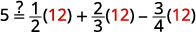##### 练习$$\PageIndex{10A}$$

$$x=12$$

##### 练习$$\PageIndex{10B}$$

$$u=−12$$

##### 示例$$\PageIndex{11}$$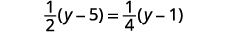分发。简化。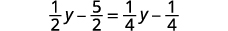乘以液晶屏，四。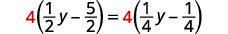分发。简化。收集左边的变量。简化。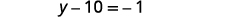收集右边的常量。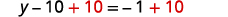简化。求解这个方程的另一种方法是清除分数而不先分布。 如果正确地将因子相乘，则此方法会更容易。乘以液晶屏，$$4$$。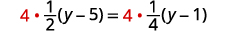将分数乘以四倍。分发。收集左边的变量。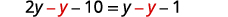简化。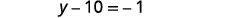收集右边的常量。简化。查看：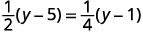让$$y=9$$。自己完成支票。
##### 练习$$\PageIndex{11A}$$

$$n=2$$

##### 练习$$\PageIndex{11B}$$

$$m=−1$$

##### 示例$$\PageIndex{12}$$

 $$\frac{4 q+3}{2}+6=\frac{3 q+5}{4}$$ 将两边乘以液晶屏，$$4$$。分发。简化。 $$2(4 q+3)+24=3 q+5$$ $$8 q+6+24=3 q+5$$ $$8 q+30=3 q+5$$ 收集左边的变量。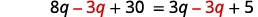简化。 $$5 q+30=5$$ 收集右边的常量。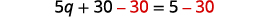简化。 $$5 q=-25$$ 将两边除以五。简化。 $$q=-5$$ 查看： $$\frac{4 q+3}{2}+6=\frac{3 q+5}{4}$$ 让$$q=−5.$$自己完成支票。

$$r=3$$

##### 练习$$\PageIndex{12B}$$

$$s=−8$$

$0.25=\frac{25}{100}, \; \; \; \;\;\;\;\; 0.05=\frac{5}{100}, \;\;\;\;\;\;\;\; 2.85=2\frac{85}{100}.$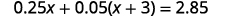先分发。将相似的术语组合在一起。要清除小数，请乘以$$100$$。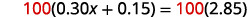分发。$$15$$从两边减去。简化。除以$$30$$。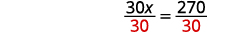简化。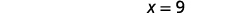代$$x=9$$入原始方程式，自己检查一下。
##### 练习$$\PageIndex{13A}$$

$$n=9$$

##### 练习$$\PageIndex{13B}$$

$$d=16$$

## 关键概念

• 如何确定一个数字是否是方程的解
1. 用数字代替方程中的变量。
2. 简化方程两边的表达式。
3. 确定所得方程是否正确。

如果是真的，那么这个数字就是一个解。

如果不是，则该数字不是解决方案。

• 如何使用通用策略求解线性方程
1. 尽可能简化方程的每一面。

使用分布属性删除所有括号。

将相似的术语组合在一起。

2. 收集方程一侧的所有变量项。

使用等式的加法或减法属性。

3. 收集方程另一侧的所有常量项。

使用等式的加法或减法属性。

4. 使变量项的系数等于 1。

使用等式的乘法或除法属性。

陈述方程的解。

5. 检查解决方案。

将解替换为原始方程以确保结果是真实的陈述。

• 如何用分数或十进制系数求解方程
1. 找出方程中所有分数和小数（以分数形式）中的最小公分母 (LCD)。
2. 将方程的两边乘以该液晶屏。 这会清除分数和小数。
3. 使用求解线性方程的通用策略进行求解。